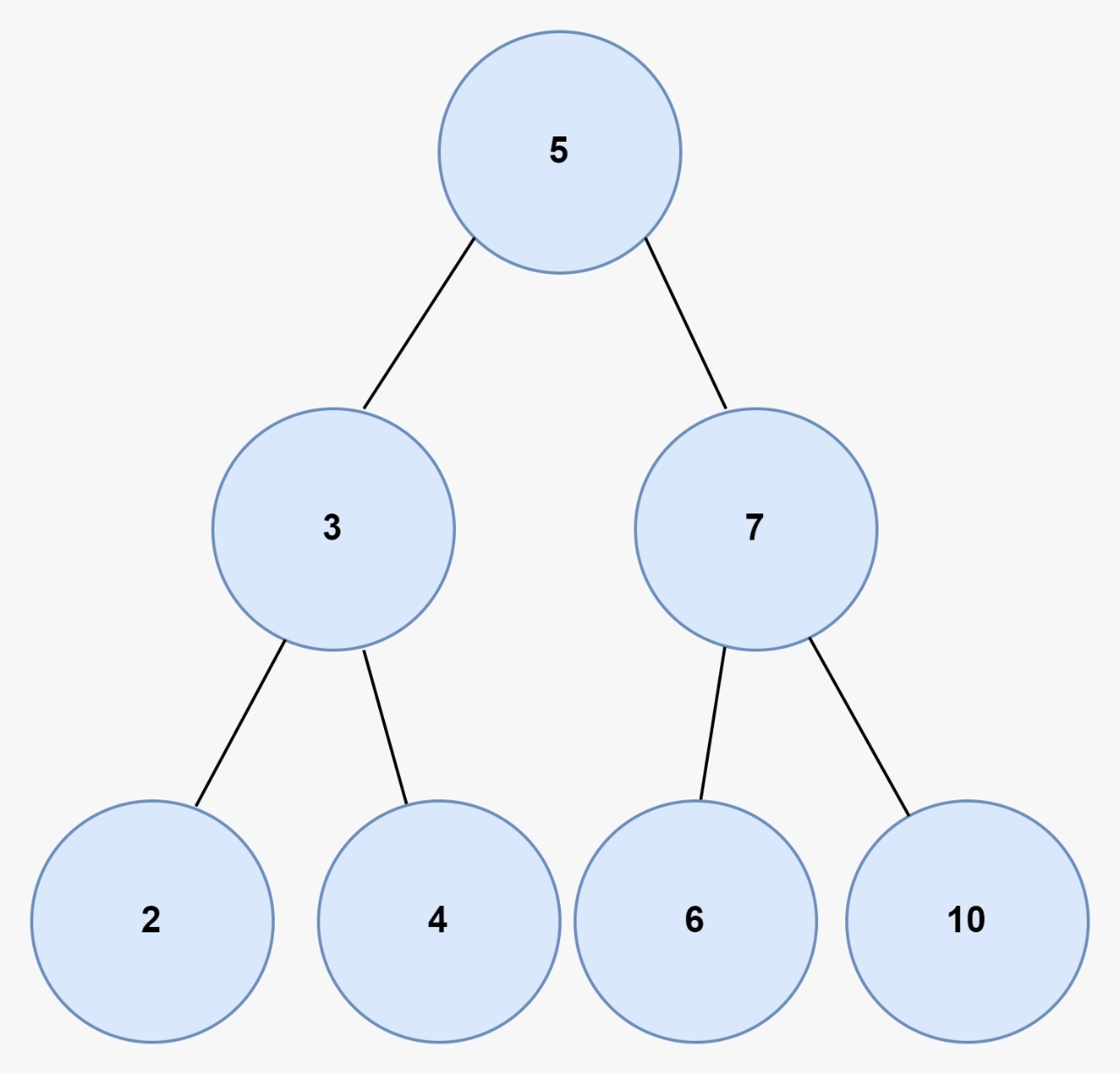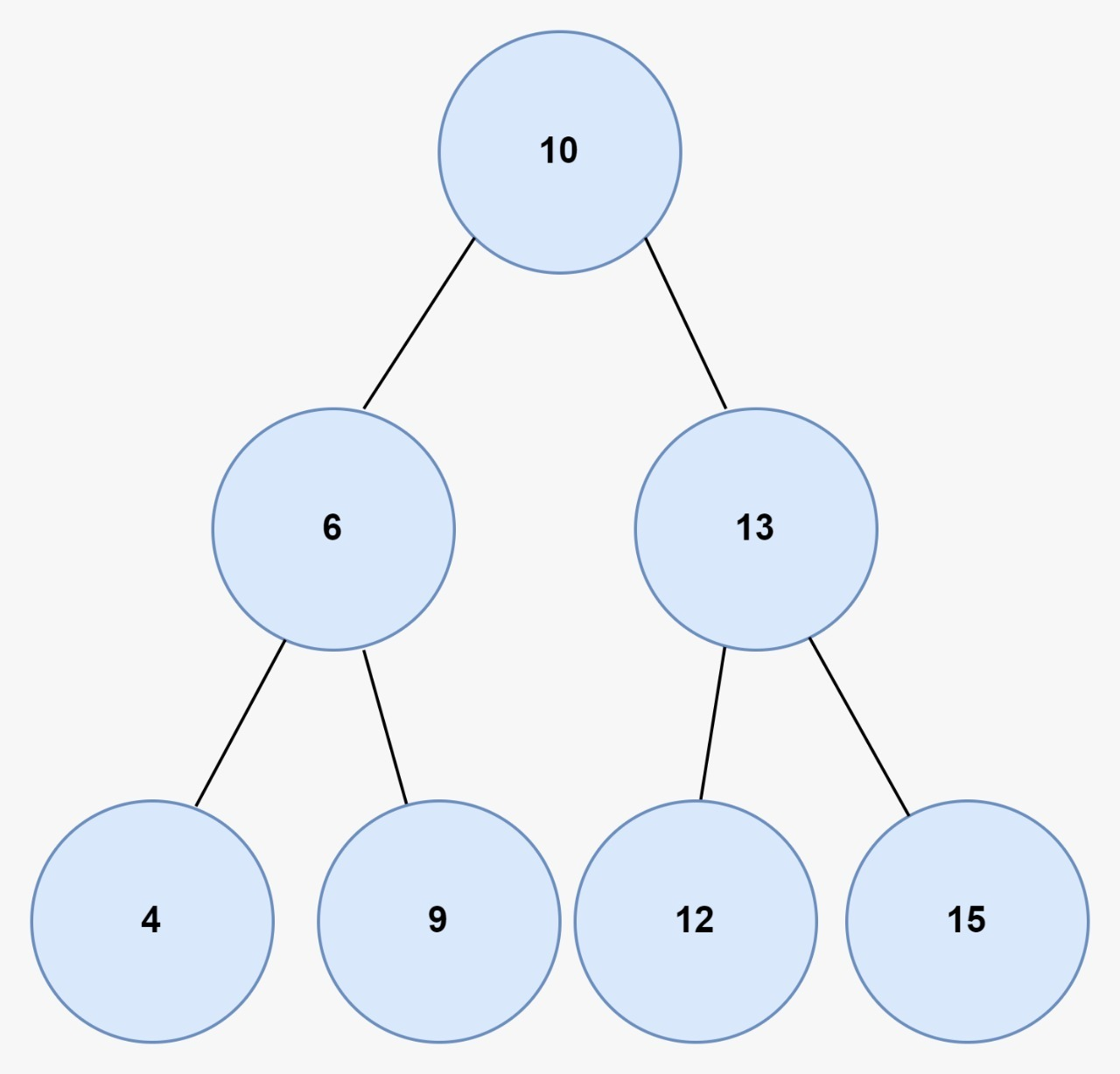# Print all pairs from two BSTs whose sum is greater than the given value

## Introduction

This blog will discuss the solution to the problem to print all pairs from two BSTs whose sum is greater than the given value where we are given two binary search trees, and we have to print all the pairs whose sum is greater than X. To understand  the problem better, look at the example given below:

Bst 1:Bst 2:X = 18

Output:

The pairs are:

{10, 10}

{10, 13}

{10, 12}

{10, 15}

{7, 13}

{7, 12}

{7, 15}

{6, 13}

{6, 15}

{5, 13}

{5, 15}

## Brute force Approach

To solve this problem, print all pairs from two BSTs whose sum is greater than the given value, we will traverse the first bst, and for every node in the first bst with value Y, we will check if a node with a value greater than (X - Y) is present in the other bst or not. If it is present, then we will print that pair.

### Algorithm

1. Create two binary search trees and pass their heads in one function named printPairs().
2. Do preorder traversal for the first tree, and every node of the first tree check for pairs in the second tree by doing preorder in the second tree.
3. If there is a pair where the sum of nodes of both the trees is greater than x, print the pair.
4. After that, make two recursive calls, one for the second binary search tree's left side and another for the second binary search tree, and another for the right side of the second binary search tree.
5. Now all the pairs will be printed one by one.

### Code in C++

``````// C++ implementation to print all pairs from two BSTs whose sum is greater than the given value
#include <bits/stdc++.h>
using namespace std;

// Structure of each node of BST
struct node
{
int key;
struct node *left, *right;
};

// Function to create a new BST node
node *TreeNode(int data)
{
node *temp = new node();
temp->key = data;
temp->left = temp->right = NULL;
return temp;
}

// function to insert node in bst
struct node *insert(struct node *node,
int key)
{
// If the tree is empty, then return a new node
if (node == NULL)
return TreeNode(key);

// Otherwise, recur down the tree
if (key < node->key)
node->left = insert(node->left,key);
else if (key > node->key)
node->right = insert(node->right,key);

// Return the (unchanged) node pointer
return node;
}
void findPair(node *root1, node *root2, int x)
{
// base case
if (!root2)
return;
// following preorder root --> left --> right
// check if the current node values of both the trees is greater than x or not
if (root1->key + root2->key > x)
{
// if greater then print the pair
cout << root1->key << " " << root2->key << endl;
}
// make recusive call to left side of second tree when it is not null
findPair(root1, root2->left, x);
// make recusive call to right side of second tree when it is not null
findPair(root1, root2->right, x);
return;
}
void printPairs(node *root1, node *root2, int x)
{
// base case
if (!root1)
return;
// following preorder root --> left --> right
// check for pairs with current node of first tree
findPair(root1, root2, x);
// make recursive call to the left side of first tree when it is not null
printPairs(root1->left, root2, x);
// make recursive call to the right side of first tree when it is not null
printPairs(root1->right, root2, x);
return;
}
int main()
{

/* Formation of BST 1
5
/ \
3   7
/\   /\
2  4 6  8
*/

struct node *root1 = NULL;
root1 = insert(root1, 5);
insert(root1, 3);
insert(root1, 2);
insert(root1, 4);
insert(root1, 7);
insert(root1, 6);
insert(root1, 8);

/* Formation of BST 2
4
/ \
2   6
/\   /\
1  3 5  7
*/

struct node *root2 = NULL;
root2 = insert(root2, 4);
insert(root2, 2);
insert(root2, 1);
insert(root2, 3);
insert(root2, 6);
insert(root2, 5);
insert(root2, 7);

int x = 10;

// Print pairs
printPairs(root1, root2, x);

return 0;
}``````

Output:

``````5 6
5 7
4 7
7 4
7 6
7 5
7 7
6 6
6 5
6 7
8 4
8 3
8 6
8 5
8 7``````

#### Complexity Analysis

Time Complexity: O(n1*h2)

Here n1 is the number of nodes in the first bst, and h2 is the height of the second tree, and since we are traversing the second tree for every node of the first tree, the time complexity is O(n1*h2).

Space Complexity: O(1)

Since no extra space is used, the space complexity will be O(1).

## Optimized Approach

In this approach, we will first traverse the first bst from the smallest node value to the largest node value, and this can be done using inorder traversal, and we will store this in an array named inorderBst1. After that, we will traverse the second bst from the largest node value to the smallest node value, and this can also be done using inorder traversal, and we will store this in another array named inorderBst2. We will take the sum of the node values from both the BST's simultaneously and traverse in both the arrays. In the first array, we will traverse using index i, and in the other array, we will traverse using index j. Now, sum = inorderBst1[i] + inorderBst2[j]. If sum > x, we will print the pair and decrement the index value j; otherwise, we will increment the index value i.

### Algorithm

1. We will first traverse bst one from smallest value node to largest value node, and we will store this inorder traversal in the array named inorderBst1 with an index called i.
2. We will traverse through the bst two from largest value node to smallest value node, and we will store this inorder traversal in an array named inorderBst2 with index named j.
3. Now we will sum the corresponding node's value from both the Bsts and check if the sum is greater than x or not.
4. If it is greater than x, we will print the pair and decrement the index j by one else; we will increment the index i by 1.

### Code in C++

``````// C++ implementation to print all pairs from two BSTs whose sum is greater than the given value
#include <bits/stdc++.h>
using namespace std;

// structure of each node of BST
struct node
{
int key;
struct node *left, *right;
};

// function to create a new BST node
node *TreeNode(int data)
{
node *temp = new node();
temp->key = data;
temp->left = temp->right = NULL;
return temp;
}

// function to insert node in bst
struct node *insert(struct node *node, int key)
{
// if the tree is empty, then return a new node
if (node == NULL)
return TreeNode(key);

// otherwise, recur down the tree
if (key < node->key)
node->left = insert(node->left, key);
else if (key > node->key)
node->right = insert(node->right, key);

// return the (unchanged) node pointer
return node;
}

// to get the size of the tree
int sizeOfTree(node *root)
{
if (root == NULL)
{
return 0;
}

// recursive call to store the left side size of the bst
int left = sizeOfTree(root->left);

// recusive call to store the right side size of the bst
int right = sizeOfTree(root->right);

// recursive call to store the total size
return (left + right + 1);
}

// function to store the inorder of the bst
void storeInorder(node *root, int inOrder[], int &index)
{
// Base condition
if (root == NULL)
{
return;
}

// left recursive call
storeInorder(root->left, inOrder, index);

// to store the elements in the array
inOrder[index++] = root->key;

// right recursive call
storeInorder(root->right, inOrder, index);
}

// function to print the pairs
void print(int inOrder[], int i, int index, int value)
{
while (i < index)
{
cout << "{" << inOrder[i] << ", " << value << "}" << endl;
i++;
}
}

// to check a valid pair and to print it
void printPairs(int inOrderBst1[], int inOrderBst2[], int index, int j, int x)
{
int i = 0;

while (i < index && j >= 0)
{

if (inOrderBst1[i] + inOrderBst2[j] > x)
{
print(inOrderBst1, i, index, inOrderBst2[j]);
j--;
}
else
{
i++;
}
}
}

// function to generate all the pairs and to print valid pairs
void checkPairs(node *root1, node *root2, int k)
{
// storing the size of first bst
int sizeOfBst = sizeOfTree(root1);

// to store the inorder of first bst
int inOrderBst1[sizeOfBst + 1];
int index1 = 0;

// storing the size of second bst
sizeOfBst = sizeOfTree(root2);

// to store the inorder of second bst
int inOrderBst2[sizeOfBst + 1];
int index2 = 0;

// to store the inorder the first bst
storeInorder(root1, inOrderBst1, index1);

// to store the inorder the second bst
storeInorder(root2, inOrderBst2, index2);

// to print the pair
printPairs(inOrderBst1, inOrderBst2, index1, index2 - 1, k);
}
int main()
{

/* Formation of BST 1
5
/ \
3   7
/\   /\
2  4 6  8
*/

struct node *root1 = NULL;
root1 = insert(root1, 5);
insert(root1, 3);
insert(root1, 2);
insert(root1, 4);
insert(root1, 7);
insert(root1, 6);
insert(root1, 8);

/* Formation of BST 2
10
/  \
8   12
/\   /\
6  9 11 13
*/

struct node *root2 = NULL;
root2 = insert(root2, 10);
insert(root2, 8);
insert(root2, 6);
insert(root2, 9);
insert(root2, 12);
insert(root2, 11);
insert(root2, 13);

int x = 18;

// print pairs
checkPairs(root1, root2, x);

return 0;
}``````

Output:

``````{6, 13}
{7, 13}
{8, 13}
{7, 12}
{8, 12}
{8, 11}``````

#### Complexity Analysis

Time Complexity: O(n1+n2)

The time complexity is O(n1+n2).

Space Complexity: O(n1 + n2)

Since we have made two arrays to store both the bst’s, the space complexity will be O(1).

Q1. What is a binary tree?

Ans. A binary tree is a data structure in programming languages to represent the hierarchy.  Each node of the binary tree can have either 0,1, or 2 children.

Q2. What are leave nodes in a tree?

Ans. Leave nodes of a tree are those nodes that don’t have any children, and both the pointers of the nodes point to null.

Q3. What is a binary search tree?

Ans. A binary search tree is a sorted binary tree in which all the nodes of the binary tree have a value greater than their left child node and a value smaller than their right child node.

## Key takeaways

In this article, we discussed the problem print all pairs from two BSTs whose sum is greater than the given value. We have discussed its two approaches: the brute force approach and the optimized approach, which is more efficient than the brute force approach in both the time and the space complexity. We hope you have gained a better understanding of the solution to this problem and, now it is your responsibility to solve the problem and try some new and different approaches to this problem.

You can learn more about binary search trees hereUntil then, Keep Learning and Keep Coding and practicing on Code studio.